### 大众(进口) 辉腾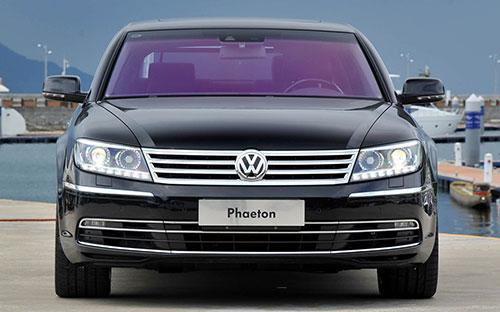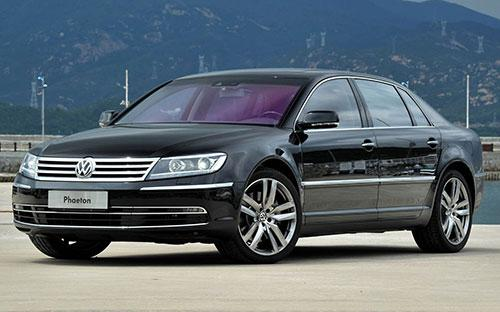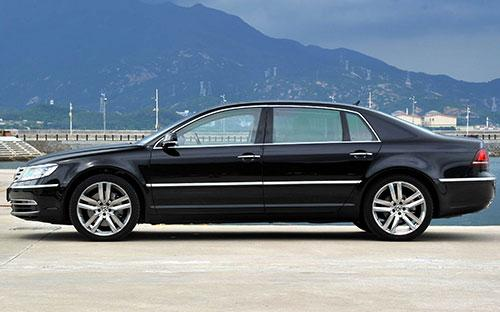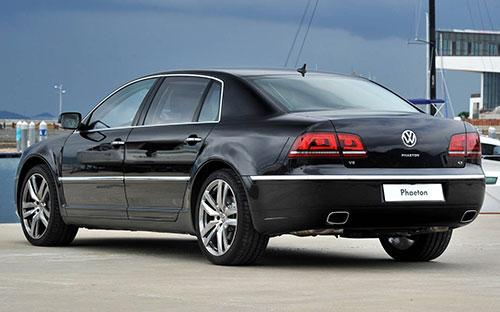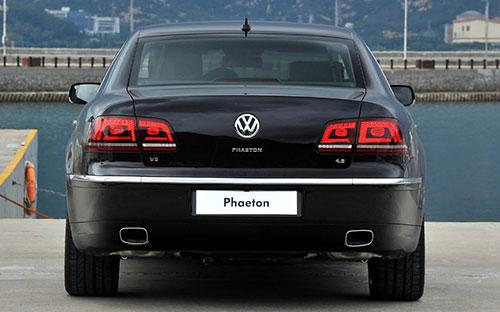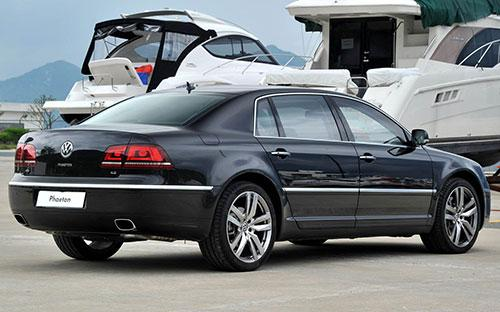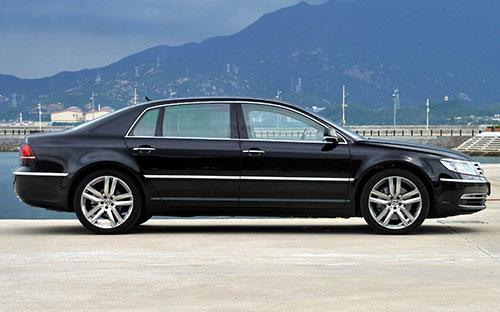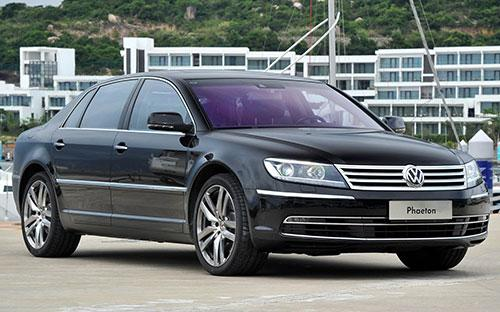10 种颜色可选2015款最低售价：79.58 万元起

5179(mm)1903(mm)1450(mm)##### 配置亮点：
• 胎压监测装置

• ISOFIX儿童座椅接口

• 车身稳定控制(ESC/ESP/DSC等)

• 电动天窗

• 定速巡航

• 后倒车雷达

• 真皮座椅

• GPS导航系统

• 氙气大灯

• 后视镜加热

• 提交
2015款 4.2L 奢享定制型 (225张)

大众(进口) 辉腾 绕车实拍• 大众(进口) 辉腾 在售车型

排量 车型 厂商指导价 本地最低报价 购车工具
3.0L
3.0L 商务型 6挡手自一体
79.58万
79.58万

3.0L 精英定制型 6挡手自一体
88.68万
88.68万

3.6L 尊享定制型 6挡手自一体
99.88万
99.88万

4.2L 奢享定制型 6挡手自一体
149.98万
149.98万

3.6L
3.0L 商务型 6挡手自一体
79.58万
79.58万

3.0L 精英定制型 6挡手自一体
88.68万
88.68万

3.6L 尊享定制型 6挡手自一体
99.88万
99.88万

4.2L 奢享定制型 6挡手自一体
149.98万
149.98万

4.2L
3.0L 商务型 6挡手自一体
79.58万
79.58万

3.0L 精英定制型 6挡手自一体
88.68万
88.68万

3.6L 尊享定制型 6挡手自一体
99.88万
99.88万

4.2L 奢享定制型 6挡手自一体
149.98万
149.98万

大众(进口) 辉腾 经销商

查看更多 >>

### 大众(进口) 辉腾 动力加速

辉腾 0-100公里加速时间分布在 6.9-10.2秒 属于 高性能级

动力级别 加速时间 车型
民用级(1款)
10.2s
3.0L 商务型3.0L 精英定制型
运动级(1款)
8.9s
3.6L 尊享定制型
高性能级(1款)
6.9s
4.2L 奢享定制型

大众(进口) 辉腾 视频

大众(进口) 辉腾 新闻资讯

# 宝马2系GranTourer奔驰C级旅行车等多款将上市深港澳车展

车系文章 超过11051次关注

北京时间6月6日上午，2015深港澳车展将正式开幕。在此次深港澳车展上，将有多款新车首发和上市，其中包括了比亚迪唐、宝马2系GranTourer、丰田新埃尔法、奔驰C级旅...

# 外观小改 大众新款辉腾将于6月6日上市

进口新车 超过9882次关注

近日我们从官方渠道获悉，大众2015款辉腾将于6月6日开幕的深港澳车展上市。据我们了解，新款车型主要针对外形设计进行了改进。

# 本周豪华车降价排行 辉腾降41.32万元

评测 超过7291次关注

时间已经到了年中，我们即将开启下半年的生活。对于爱车的您来讲，不知道有没有计划在年中时期购置一辆喜欢的车型。今天，我们将带给您的是豪华车降价排行榜，里面...

# 进口大众多款车搭载车联网系统 4年免费

技术 超过7252次关注

大众进口汽车正式推出了车联网服务。该服务将搭载于进口Tiguan、甲壳虫、尚酷、高尔夫R敞篷轿车、夏朗、迈腾旅行轿车、迈腾四驱旅行车等多款大众进口车型上，并为用...

# 大众40余款车型价格上调 最高涨2.84万

文化 超过0次关注

近日我们从大众中国了解到，它们上调了一部分进口车型的市场指导价格，涉及包括甲壳虫、迈腾旅行版、途锐、夏朗以及Tiguan等车型，最高涨幅也达到了2.84万元。

# 新一代辉腾最快2017年发布 定位或调整

新闻 超过7350次关注

日前，海外媒体曝光了一组新一代辉腾的信息，新车最快将会在2017年发布。

# 大众全新一代辉腾假想图 或2018年推出

进口新车 超过6156次关注

近日，海外媒体曝光了一张大众全新一代辉腾车型的假想图，据了解，这款新车或将在2017年发布，随后在2018年推出。

猜你喜欢

﻿
• 快速找车
• 选择品牌
• 选择品牌
• A  奥迪
• A  阿斯顿·马丁
• A  阿尔法·罗密欧
• B  宝沃
• B  布加迪
• B  巴博斯
• B  保时捷
• B  宾利
• B  奔驰
• B  宝马
• B  本田
• B  别克
• B  标致
• B  比亚迪
• B  宝骏
• B  北汽制造
• B  北汽新能源
• B  北汽幻速
• B  北汽威旺
• B  北京汽车
• B  奔腾
• B  北汽绅宝
• C  长安
• C  长安商用
• C  长城
• C  昌河
• D  大众
• D  道奇
• D  DS
• D  东南
• D  东风风神
• D  东风风行
• D  东风小康
• D  东风风度
• D  东风
• F  福特
• F  丰田
• F  菲亚特
• F  法拉利
• F  福田
• F  福迪
• F  福汽启腾
• G  观致
• G  广汽传祺
• G  广汽吉奥
• G  GMC
• H  红旗
• H  汉腾汽车
• H  哈弗
• H  哈飞
• H  海格
• H  海马
• H  华颂
• H  黄海
• H  华泰
• H  恒天
• J  吉利汽车
• J  捷豹
• J  Jeep
• J  江淮
• J  江铃
• J  金杯
• J  九龙
• J  金旅
• K  凯翼
• K  凯迪拉克
• K  克莱斯勒
• K  科尼塞克
• K  卡威
• K  开瑞
• L  路虎
• L  林肯
• L  劳斯莱斯
• L  兰博基尼
• L  雷克萨斯
• L  铃木
• L  雷诺
• L  理念
• L  力帆
• L  莲花汽车
• L  猎豹
• L  路特斯
• L  陆风
• M  马自达
• M  MG
• M  MINI
• M  玛莎拉蒂
• M  摩根
• M  迈凯轮
• N  纳智捷
• O  欧宝
• O  讴歌
• O  欧朗
• Q  奇瑞
• Q  起亚
• Q  启辰
• R  日产
• R  荣威
• R  瑞麒
• S  三菱
• S  斯威汽车
• S  萨博
• S  smart
• S  斯柯达
• S  斯巴鲁
• S  思铭
• S  双龙
• S  上汽大通
• S  双环
• T  特斯拉
• T  腾势
• W  沃尔沃
• W  五菱汽车
• W  五十铃
• W  威兹曼
• W  威麟
• X  现代
• X  雪佛兰
• X  雪铁龙
• X  西雅特
• Y  一汽
• Y  英菲尼迪
• Y  英致
• Y  依维柯
• Y  野马汽车
• Y  永源
• Z  众泰
• Z  中华
• Z  中兴
• Z  知豆
• 选择车系
• 选择车系
• 车型对比
• 选择品牌
• 选择品牌
• A  奥迪
• A  阿斯顿·马丁
• A  阿尔法·罗密欧
• B  宝沃
• B  布加迪
• B  巴博斯
• B  保时捷
• B  宾利
• B  奔驰
• B  宝马
• B  本田
• B  别克
• B  标致
• B  比亚迪
• B  宝骏
• B  北汽制造
• B  北汽新能源
• B  北汽幻速
• B  北汽威旺
• B  北京汽车
• B  奔腾
• B  北汽绅宝
• C  长安
• C  长安商用
• C  长城
• C  昌河
• D  大众
• D  道奇
• D  DS
• D  东南
• D  东风风神
• D  东风风行
• D  东风小康
• D  东风风度
• D  东风
• F  福特
• F  丰田
• F  菲亚特
• F  法拉利
• F  福田
• F  福迪
• F  福汽启腾
• G  观致
• G  广汽传祺
• G  广汽吉奥
• G  GMC
• H  红旗
• H  汉腾汽车
• H  哈弗
• H  哈飞
• H  海格
• H  海马
• H  华颂
• H  黄海
• H  华泰
• H  恒天
• J  吉利汽车
• J  捷豹
• J  Jeep
• J  江淮
• J  江铃
• J  金杯
• J  九龙
• J  金旅
• K  凯翼
• K  凯迪拉克
• K  克莱斯勒
• K  科尼塞克
• K  卡威
• K  开瑞
• L  路虎
• L  林肯
• L  劳斯莱斯
• L  兰博基尼
• L  雷克萨斯
• L  铃木
• L  雷诺
• L  理念
• L  力帆
• L  莲花汽车
• L  猎豹
• L  路特斯
• L  陆风
• M  马自达
• M  MG
• M  MINI
• M  玛莎拉蒂
• M  摩根
• M  迈凯轮
• N  纳智捷
• O  欧宝
• O  讴歌
• O  欧朗
• Q  奇瑞
• Q  起亚
• Q  启辰
• R  日产
• R  荣威
• R  瑞麒
• S  三菱
• S  斯威汽车
• S  萨博
• S  smart
• S  斯柯达
• S  斯巴鲁
• S  思铭
• S  双龙
• S  上汽大通
• S  双环
• T  特斯拉
• T  腾势
• W  沃尔沃
• W  五菱汽车
• W  五十铃
• W  威兹曼
• W  威麟
• X  现代
• X  雪佛兰
• X  雪铁龙
• X  西雅特
• Y  一汽
• Y  英菲尼迪
• Y  英致
• Y  依维柯
• Y  野马汽车
• Y  永源
• Z  众泰
• Z  中华
• Z  中兴
• Z  知豆
• 选择车系
• 选择车系
• 选择车型
• 选择车型
• 意见反馈